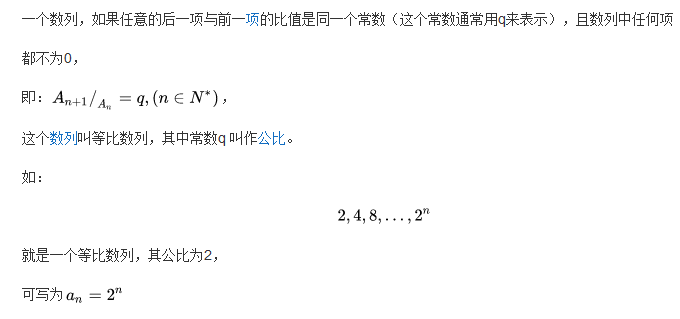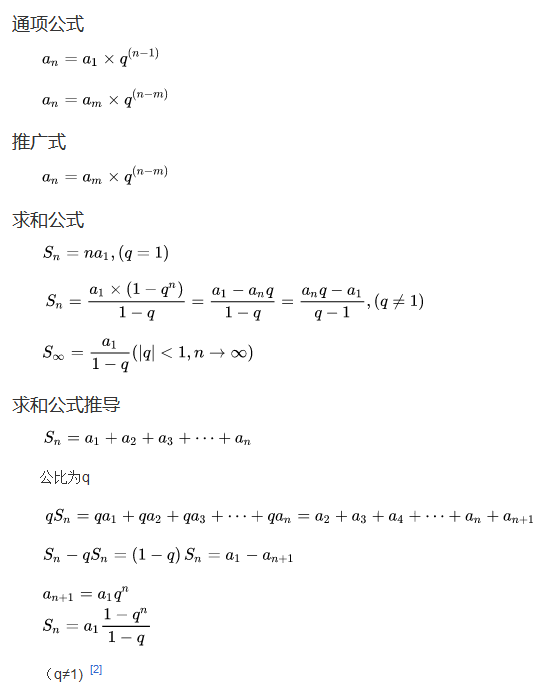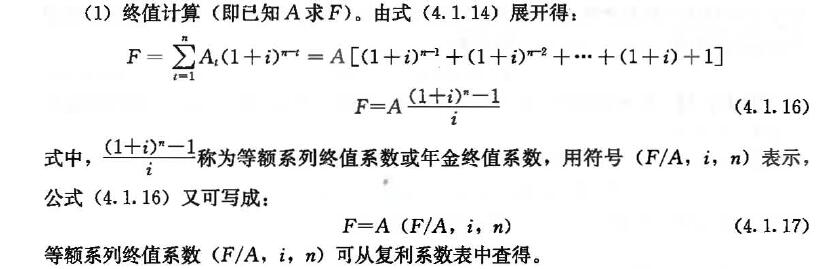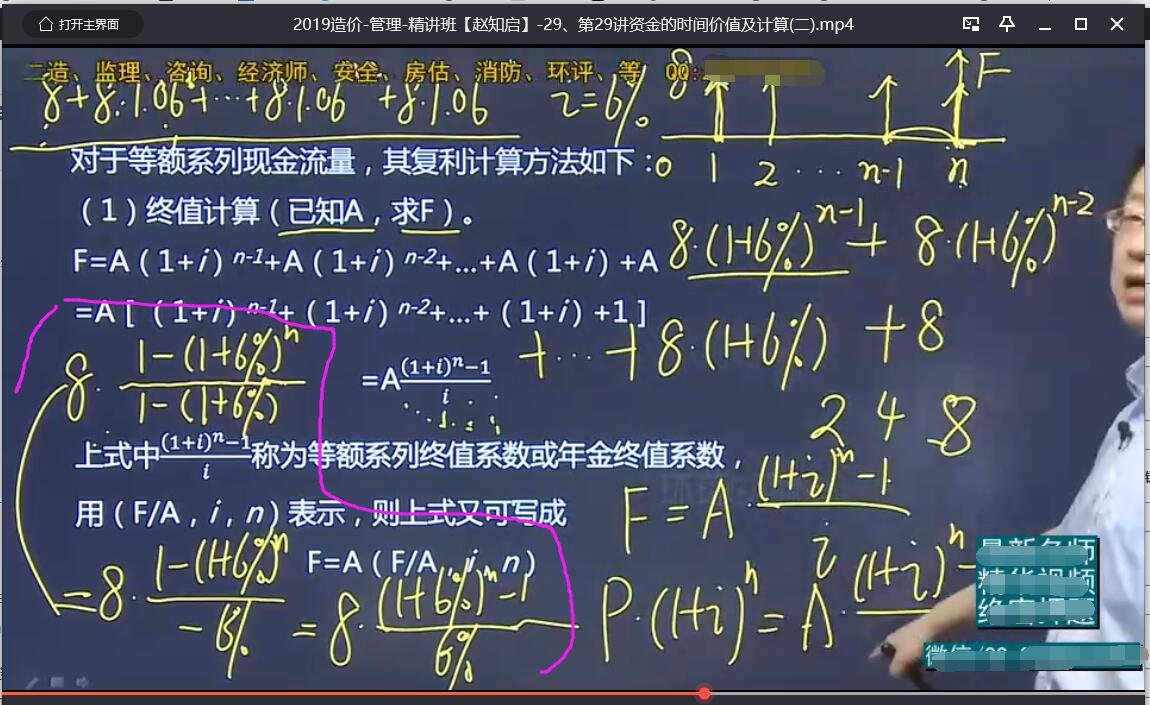# 等比数列求和公式推导普通年金终值计算公式

2020/07/26

1,1602

#### 等比数列求和公式

##### 定义##### 公式#### 用等比数列求和公式推导普通年金终值计算公式

(1)期首付。首次支付在0时刻，到n年末年复利计息本利和为a(1+i)^n，第二次支付在1时刻，期末累积n-1次，本利和a(1+i)^(n-1),…,第n次支付在n-1时刻，累积1次，本利和a(1+i)。

∴ 所付年金总额Sn=a(1+i)^n+a(1+i)^(n-1)+…+a(1+i)【按递增顺序】构成首项a(1+i)、公比(1+i)等比数列。

Sn两边同乘以(1+i)后相减,有(1+i)Sn-Sn=a(1+i)^(n+1)-a(1+i)。

∴ Sn=a[(1+i)^n-1]/d       d=i/(1+i)。

(2)期末付。首次支付在1时刻,到n年末年复利计息的本利和为a(1+i)^(n-1),第二次支付在2时刻，期末累积n-2次,本利和a(1+i)^(n-2),…,第n次支付在n时刻，本利和a。

∴所付年金总额仿照(1)的计算,得Sn=a[(1+i)^n-1]/i。供参考。